Courses

# NFA to DFA Conversion Computer Science Engineering (CSE) Notes | EduRev

## Computer Science Engineering (CSE) : NFA to DFA Conversion Computer Science Engineering (CSE) Notes | EduRev

The document NFA to DFA Conversion Computer Science Engineering (CSE) Notes | EduRev is a part of the Computer Science Engineering (CSE) Course Theory of Computation.
All you need of Computer Science Engineering (CSE) at this link: Computer Science Engineering (CSE)

Part III. NFA to DFA Conversion

Consider the following NFA,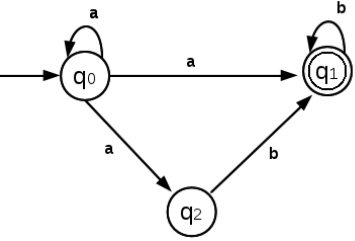In the above NFA, from state q0, there are 3 possible moves for input symbol, a. ie.. to q0, q1, q2. When we simulate this NFA using a program, the move from q0 for symbol a is non-deterministic. That means, which transition is to be made next cannot be determined in one move. But in a DFA, from a state, for an input symbol, only one transition exists. So it is very easy to simulate a DFA using a program. So we need to convert an NFA to an equivalent DFA. For every NFA, there exists an equivalent DFA.

5 Epsilon Closure
For converting an NFA to DFA, we first need to learn how to find the epsilon closure of a state.
Epsilon closure (ε- closure) of a state q is the set that contains the state q itself and all other states that can be reached
from state q by following ε transitions.
We use the term, ECLOSE( q0) to denote the Epsilon closure of state q0.
For example,
Example 1:
Consider the following NFA,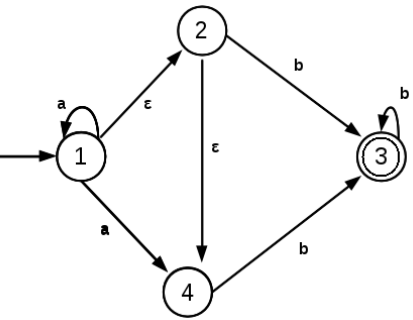Find the epsilon closure of all states.
Here,
ECLOSE(1) = {1, 2, 4}
ECLOSE(2) = {2, 4}
ECLOSE(3) = {3}
ECLOSE(4) = {4}

Example 2:
Consider the following NFA,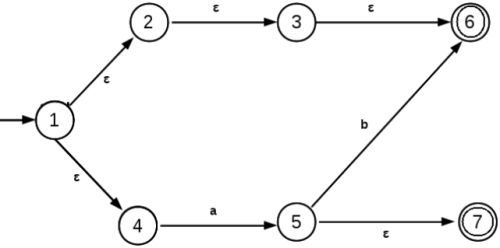Find the epsilon closure of all states.
ECLOSE(1) = {1, 2, 3, 6, 4}
ECLOSE(2) = {2, 3, 6}
ECLOSE(3) = {3, 6}
ECLOSE(4) = {4}
ECLOSE(5) = {5, 7}
ECLOSE(6) = {6}

Example 3:
Consider the following NFA,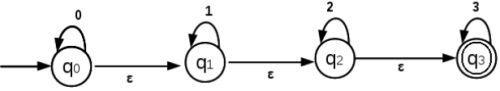Compute the Epsilon closure of all states.
ECLOSE(q0) = {q0, q1, q2, q3}
ECLOSE(q1) = {q1, q2, q3}
ECLOSE(q2) = {q2, q3}
ECLOSE(q3) = {q3}

6 Conversion of NFA to DFA
The steps involved in the conversion of NFA to DFA are,
1. Transform the NFA with Epsilon transitions to NFA without epsilon transitions.
2. convert the resulting NFA to DFA.
These steps are exaplained in detail as follows:

1. Transform the NFA with ε transitions to NFA without ε transitions.
Example 1:
Consider the following NFA with epsilon transitions: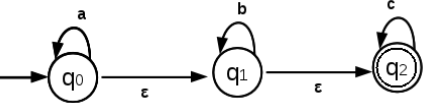The above NFA has states, q0, q1, q2. The start state is q0. The final state is q2.
Step a: Find the Epsion closure of all states.
ECLOSE(q0) = {q0, q1, q2}
ECLOSE(q1) = { q1, q2}
ECLOSE(q2) = {q2}
The states of the new NFA will be {q0, q1, q2}, {q1, q2}, {q2}.
The start state of the new NFA will be the epsilon closure of the start state q0.
Thus the start state of the new NFA will be {q0, q1, q2}
The final states of the new NFA will be those new states that contain the final states of the old NFA.
Thus the final states of the new NFA are {q0, q1, q2}, {q1, q2}, {q2}, since they contain the final state q2 of the old NFA.
Next we need to find the transitions of these new states on input symbols a, b and c.
Consider state {q0, q1, q2},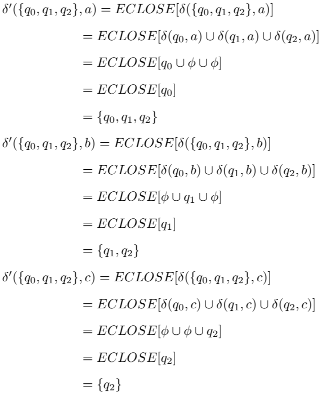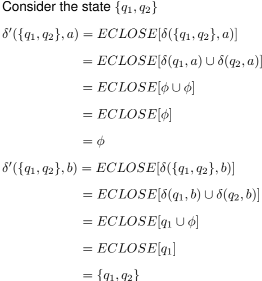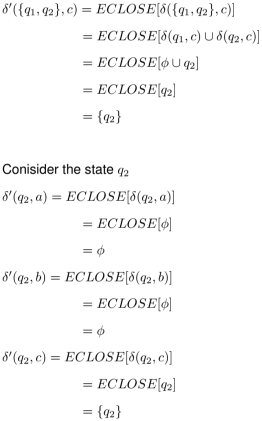Now we have the new NFA without epsilon transitions.
The transition table for the new NFA is,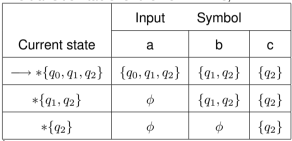Let us say,
{q0, q1, q2} as qx
{q1, q2} as qy
{q2} as qz
Then the transition table will become,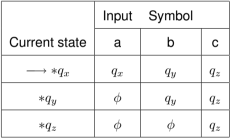The transition diagram for the new NFA is,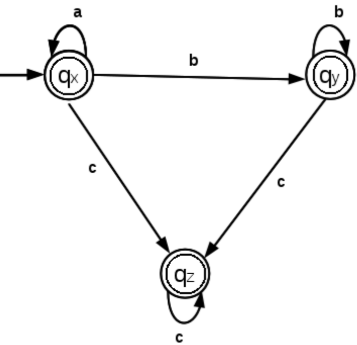Above is the NFA without epsilon transitions.
Note that above NFA is also a DFA.

Example 2:
Convert the following NFA to DFA.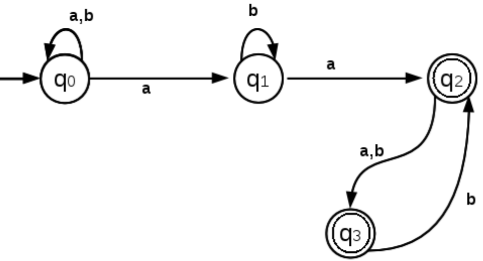Step 1: Transform the NFA with Epsilon transitions to NFA without epsilon transitions.
Note that above NFA does not contain any epsilon transitions.

Step 2: Convert the resulting NFA to DFA.
Step a:
Consider the start state q0,
Seek all the transitions from state q0 for all input symbols a and b.
We get,
δ(q0, a) = {q0, q1}
δ(q0, b) = {q0}
Now we got,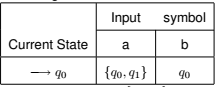Here we got a new state, {q0, q1}
Step b:
Consider the state {q0, q1},
Seek all the transitions from state {q0, q1}for all input symbols a and b.
We get,
δ({q0, q1}, a) = δ(q0, a) ∪ δ(q1, a)
= {q0, q1} ∪ q2
= {q0, q1, q2}
δ({q0, q1}, b) = δ(q0, b) ∪ δ(q1, b)
= q0 ∪ q1
= {q0, q1}
Now we got,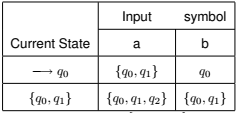Here we got a new state, {q0, q1, q2}
Step c:
Consider the state {q0, q1, q2},
Seek all the transitions from state {q0, q1, q2}for all input symbols a and b.
We get,
δ({q0, q1, q2}, a) = δ(q0, a) ∪ δ(q1, a) ∪ δ(q2, a)
= {q0, q1} ∪ q2 ∪ q3
= {q0, q1, q2, q3}
δ({q0, q1, q2}, b) = δ(q0, b) ∪ δ(q1, b) ∪ δ(q2, b)
= q0 ∪ q1  ∪ q3
= {q0, q1, q3}
Now we got,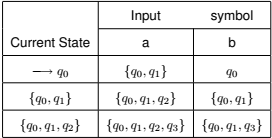Here we got 2 new states, {q0, q1, q2, q3} and {q0, q1, q3}.

Step d:
Consider each one of these states, {q0, q1, q2, q3} and {q0, q1, q3}
Step d.i:
Consider the state {q0, q1, q2, q3},
Seek all the transitions from state {q0, q1, q2, q3}for all input symbols a and b.
We get,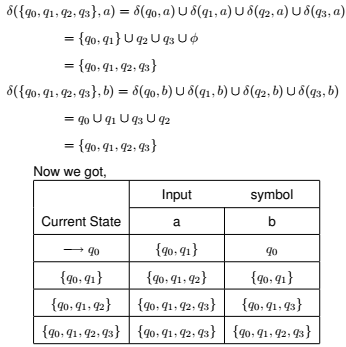Step d.i:
Consider the state {q0, q1,q3},
Seek all the transitions from state {q0, q1,q3} for all input symbols a and b.
We get,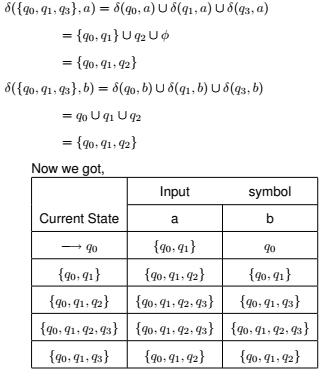Now there are no new states generated. above is the transition table corresponding to the new DFA.
In the above DFA, the start state is q0.
The final states of the DFA are {q0, q1, q2},{q0, q1, q2, q3},{q0, q1,q3}, since they contain at least one final state of the NFA
(q2 or q3).
Let us say,
qas A,
{q0, q1} as B,
{q0, q1, q2} as C,
{q0, q1, q2, q3} as D, and
{q0, q1,q3} as E
Then the transition table will become,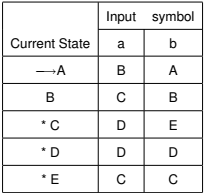The transition diagram corresponding to the DFA is,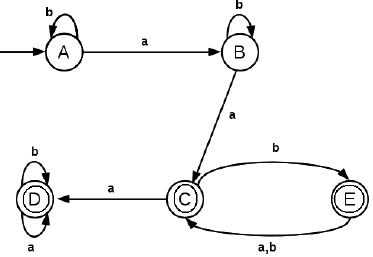From the above diagram, it is a DFA since, there is only one transition corresponding to an input symbol from a state.
Example 3:
Consider the following NFA,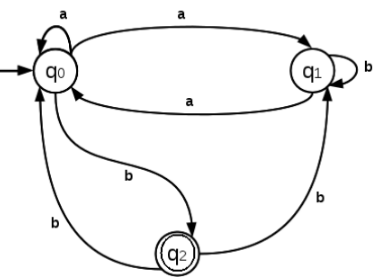Step 1: Transform the NFA with Epsilon transitions to NFA without epsilon transitions.Note that above NFA does not contain any epsilon transitions.
Step 2: Convert the resulting NFA to DFA.
Consider the start state q0,
Seek all the transitions from state q0 for all input symbols a and b.
We get,
δ(q0, a) = {q0, q1}
δ(q0, b) = {q2}
Now we got,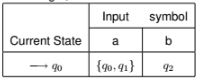Step b:
Step b.i: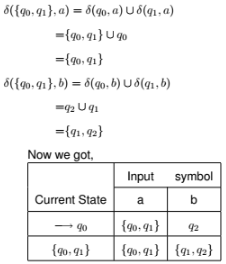Step b. i. i: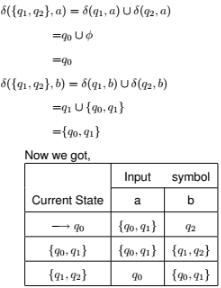Step b.ii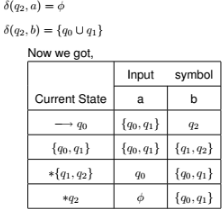Thus there are no more new states. The above is the transition table for the new NFA.
The start state is q0.
The final states are qand {q1, q2}, since they contain the final state, q2 of the NFA.
Let we say,
q0as A
{q0, q1} as B
{q1, q2} as C
q2 as D.
The new transition table is,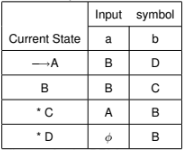The transition diagram for the DFA is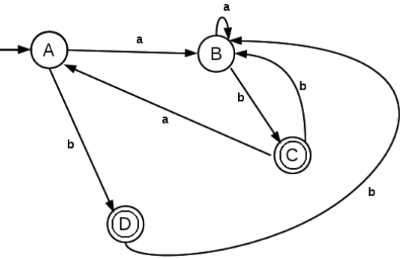Example 4:
Consider the NFA,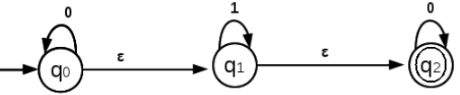Convert the above NFA to DFA.
Step 1: Transform the NFA with Epsilon transitions to NFA without epsilon transitions.
Step a:
The states of the new NFA will be ECLOSE(q0), ECLOSE(q1), ECLOSE(q2).
Find the Epsion closure of all states.
ECLOSE(q0) = {q0, q1, q2}
ECLOSE(q1) = {q1, q2}
ECLOSE(q2) = {q2}

The states of the new NFA will be {q0, q1, q2}, {q0, q1, q2}.
The start state of the new NFA will be the epsilon closure of the start state q0.
Thus the start state of the new NFA will be {q0, q1, q2}
The final states of the new NFA will be those new states that contain the final states of the old NFA.
Thus the final states of the new NFA are {q0, q1, q2}, {q1, q2}, {q2}, since they contain the final state q2 of the old NFA.

Next we need to find the transitions of these new states on input symbols 0 and 1.
Consider state {q0, q1, q2},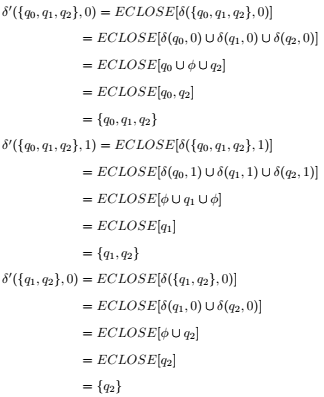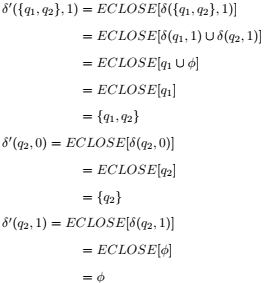Let us say,
{q0, q1, q2} as A,
{q1, q2} as B, and
{q2} as C.
Then the NFA without epsilon transitions is,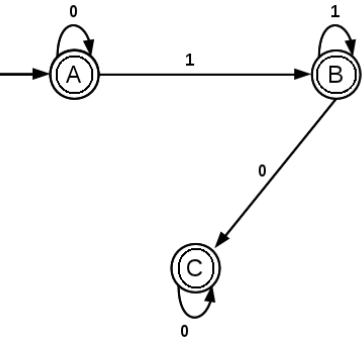The above is an NFA without epsilon transitions.

Step 2: Convert the resulting NFA to DFA.
Note that above NFA is also a DFA, since from a state there is only one transition corresponding to an input symbol.

Example 5:
Consider the following NFA,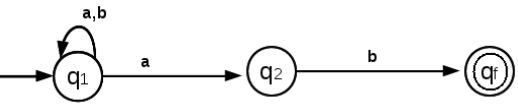Convert this NFA to DFA.

Step 1: Transform the NFA with Epsilon transitions to NFA without epsilon transitions.
Above NFA does not contain epsilon transitions. So we need to eliminate epsilon transitions.

Step 2: Transform above NFA to DFA.
Begin from the start state, q1,
δ(q1, a) = {q1, q2}
δ(q1, b) = {q1}
Now we got,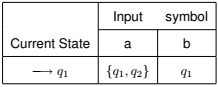Input symbol
Step b: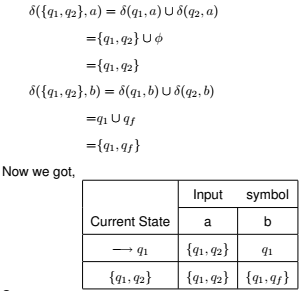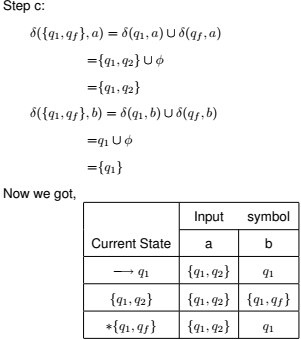The start state is q1
The final state is {q1, qf}
Let us say,
q1 as A,
{q1, q2} as B,
{q1, q} as C.
Then the transition table will become,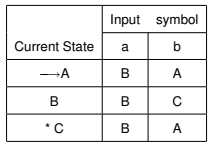The transition diagram is,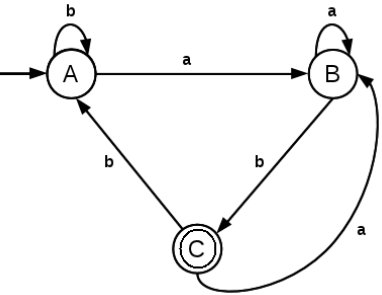The above is a DFA, since there is only one transition corresponding to an input symbol from every state.

Exercises:
1. Convert the following NFA to DFA.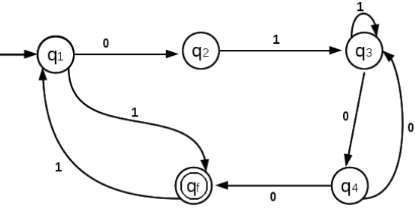2. Convert the following NFA to DFA.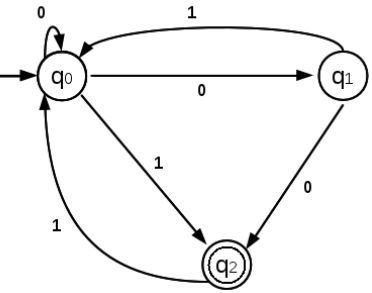3. Convert the following NFA to DFA.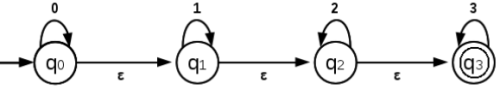Offer running on EduRev: Apply code STAYHOME200 to get INR 200 off on our premium plan EduRev Infinity!

## Theory of Computation

18 videos|43 docs|39 tests

,

,

,

,

,

,

,

,

,

,

,

,

,

,

,

,

,

,

,

,

,

;